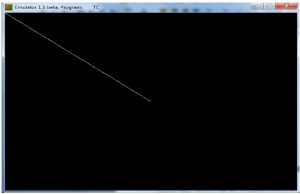# DDA Line generation Algorithm in Computer Graphics

In any 2-Dimensional plane if we connect two points (x0, y0) and (x1, y1), we get a line segment. But in the case of computer graphics we can not directly join any two coordinate points, for that we should calculate intermediate point’s coordinate and put a pixel for each intermediate point, of the desired color with help of functions like putpixel(x, y, K) in C, where (x,y) is our co-ordinate and K denotes some color.

Examples:

```Input: For line segment between (2, 2) and (6, 6) :
we need (3, 3) (4, 4) and (5, 5) as our intermediate
points.

Input: For line segment between (0, 2) and (0, 6) :
we need (0, 3) (0, 4) and (0, 5) as our intermediate
points.
```

For using graphics functions, our system output screen is treated as a coordinate system where the coordinate of the top-left corner is (0, 0) and as we move down our y-ordinate increases and as we move right our x-ordinate increases for any point (x, y).
Now, for generating any line segment we need intermediate points and for calculating them we have can use a basic algorithm called DDA(Digital differential analyzer) line generating algorithm.

DDA Algorithm :
Consider one point of the line as (X0,Y0) and the second point of the line as (X1,Y1).

```// calculate dx , dy
dx = X1 - X0;
dy = Y1 - Y0;

// Depending upon absolute value of dx & dy
// choose number of steps to put pixel as
// steps = abs(dx) > abs(dy) ? abs(dx) : abs(dy)
steps = abs(dx) > abs(dy) ? abs(dx) : abs(dy);

// calculate increment in x & y for each steps
Xinc = dx / (float) steps;
Yinc = dy / (float) steps;

// Put pixel for each step
X = X0;
Y = Y0;
for (int i = 0; i <= steps; i++)
{
putpixel (X,Y,WHITE);
X += Xinc;
Y += Yinc;
}
```

## Recommended: Please try your approach on {IDE} first, before moving on to the solution.

 `// C program for DDA line generation ` `#include ` `#include ` ` `  `//Function for finding absolute value ` `int` `abs` `(``int` `n) ` `{ ` `    ``return` `( (n>0) ? n : ( n * (-1))); ` `} ` ` `  `//DDA Function for line generation ` `void` `DDA(``int` `X0, ``int` `Y0, ``int` `X1, ``int` `Y1) ` `{ ` `    ``// calculate dx & dy ` `    ``int` `dx = X1 - X0; ` `    ``int` `dy = Y1 - Y0; ` ` `  `    ``// calculate steps required for generating pixels ` `    ``int` `steps = ``abs``(dx) > ``abs``(dy) ? ``abs``(dx) : ``abs``(dy); ` ` `  `    ``// calculate increment in x & y for each steps ` `    ``float` `Xinc = dx / (``float``) steps; ` `    ``float` `Yinc = dy / (``float``) steps; ` ` `  `    ``// Put pixel for each step ` `    ``float` `X = X0; ` `    ``float` `Y = Y0; ` `    ``for` `(``int` `i = 0; i <= steps; i++) ` `    ``{ ` `        ``putpixel (X,Y,RED);  ``// put pixel at (X,Y) ` `        ``X += Xinc;           ``// increment in x at each step ` `        ``Y += Yinc;           ``// increment in y at each step ` `        ``delay(100);          ``// for visualization of line- ` `                             ``// generation step by step ` `    ``} ` `} ` ` `  `// Driver program ` `int` `main() ` `{ ` `    ``int` `gd = DETECT, gm; ` ` `  `    ``// Initialize graphics function ` `    ``initgraph (&gd, &gm, ``""``);    ` ` `  `    ``int` `X0 = 2, Y0 = 2, X1 = 14, Y1 = 16; ` `    ``DDA(2, 2, 14, 16); ` `    ``return` `0; ` `}  `

Output:

``````

• It is simple and easy to implement algorithm.
• It avoid using multiple operations which have high time complexities.
• It is faster than the direct use of the line equation because it does not use any floating point multiplication and it calculates points on the line.

• It deals with the rounding off operation and floating point arithmetic so it has high time complexity.
• As it is orientation dependent, so it has poor endpoint accuracy.
• Due to the limited precision in the floating point representation it produces cumulative error.

Bresenham’s Line Generation Algorithm

Don’t stop now and take your learning to the next level. Learn all the important concepts of Data Structures and Algorithms with the help of the most trusted course: DSA Self Paced. Become industry ready at a student-friendly price.

My Personal Notes arrow_drop_up

Article Tags :
Practice Tags :

4

Please write to us at contribute@geeksforgeeks.org to report any issue with the above content.